Math Olympiad Test: Circles- 2

# Math Olympiad Test: Circles- 2

Test Description

## 15 Questions MCQ Test Mathematics Olympiad for Class 9 | Math Olympiad Test: Circles- 2

Math Olympiad Test: Circles- 2 for Class 9 2022 is part of Mathematics Olympiad for Class 9 preparation. The Math Olympiad Test: Circles- 2 questions and answers have been prepared according to the Class 9 exam syllabus.The Math Olympiad Test: Circles- 2 MCQs are made for Class 9 2022 Exam. Find important definitions, questions, notes, meanings, examples, exercises, MCQs and online tests for Math Olympiad Test: Circles- 2 below.
Solutions of Math Olympiad Test: Circles- 2 questions in English are available as part of our Mathematics Olympiad for Class 9 for Class 9 & Math Olympiad Test: Circles- 2 solutions in Hindi for Mathematics Olympiad for Class 9 course. Download more important topics, notes, lectures and mock test series for Class 9 Exam by signing up for free. Attempt Math Olympiad Test: Circles- 2 | 15 questions in 15 minutes | Mock test for Class 9 preparation | Free important questions MCQ to study Mathematics Olympiad for Class 9 for Class 9 Exam | Download free PDF with solutions
 1 Crore+ students have signed up on EduRev. Have you?
Math Olympiad Test: Circles- 2 - Question 1

### In the adjoining figure, O is the circumcentre of ∆ABC, then the value of ∠OBC + ∠BAC is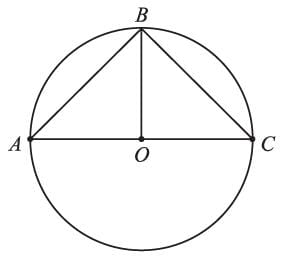Detailed Solution for Math Olympiad Test: Circles- 2 - Question 1

In ∆OAB,
OA = OB
∴ ∠OAB = ∠OBA …(i)
∵ ∠OBC + ∠OBA = ∠ABC
∠OBC + ∠BAC = ∠ABC
∠OBC + ∠BAC = 90°
[∵ ∠ABC is the angle in semicircle]

Math Olympiad Test: Circles- 2 - Question 2

### In the adjoining figure, AB = AC, and ∠ACB = 64°, then ∠BEC =?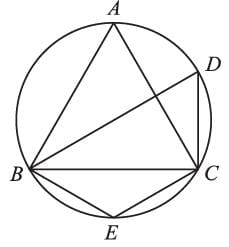Detailed Solution for Math Olympiad Test: Circles- 2 - Question 2

∵ AB = BC
∴ ∠ABC = ∠ACB = 64°
∴ ∠BAC = 180° – 64° × 2 = 52°
∴ ABCD is a cyclic quadrilateral
∴ ∠A + ∠E = 180°
∴ ∠A + ∠E = 180°
= 128°

Math Olympiad Test: Circles- 2 - Question 3

### AB || CD, and ∠B = 65° and ∠DAC = 30° The measure of ∠CAB =?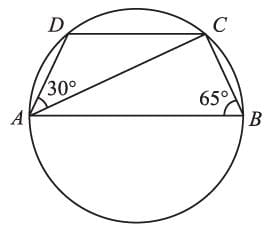Detailed Solution for Math Olympiad Test: Circles- 2 - Question 3

Let ∠CAB = x,
∴ ∠ACD = x (Alternate ∠S)
In ∆ACD,
∠D = 180° – (30° + x)
= 150° – x
∵ ABCD is a cyclic quadrilateral
∴ ∠D + ∠B = 180°
⇒ 150° – x + 65° = 180°
⇒ x = 35°

Math Olympiad Test: Circles- 2 - Question 4

In a cyclic quadrilateral, AB || CD, then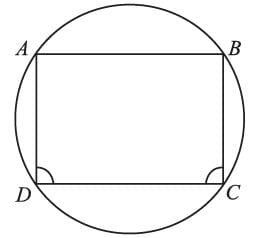Detailed Solution for Math Olympiad Test: Circles- 2 - Question 4

∵ ABCD is a cyclic quadrilateral
∴ ∠A + ∠C = ∠B + ∠D = 180° …(i)
Also,
∠A + ∠D = ∠B + ∠C = 180° …(ii)
∴     ∠B = ∠C [using (i) and (ii)]
∵ ABCD is a trapezium having ∠C = ∠D
∴ ABCD should be an isosceles trapezium
⇒ AD = BC

Math Olympiad Test: Circles- 2 - Question 5

Find x (O is the center of the circle):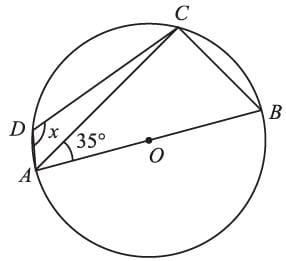Detailed Solution for Math Olympiad Test: Circles- 2 - Question 5

In ∆CBA,
∠A + ∠C + ∠B = 180°
⇒ ∠B = 180° – 35° – 90°
[∵ ∠C = 90°, i.e., angle in a semicircle]
⇒ ∠B = 55°
∵ ABCD is a cyclic quadrilateral
∴ ∠B + ∠D = 180°
⇒ x = 180° – 55°
= 125°

Math Olympiad Test: Circles- 2 - Question 6

The measures of AB and CD are equal, and the measure of ∠LOM = 160°. The measure of ∠OLM is: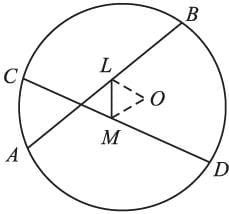Detailed Solution for Math Olympiad Test: Circles- 2 - Question 6

∵ AB = CD
∴ OL = OM, as the distance of equal chords from the center of the circle, should be equal
⇒ ∠OLM = ∠OML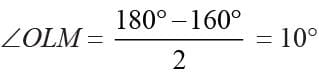Math Olympiad Test: Circles- 2 - Question 7

If the two diameters of a circle intersect at 90°. The figure formed by joining the endpoint of the diameters will be a:

Detailed Solution for Math Olympiad Test: Circles- 2 - Question 7

∵ Diagonals of the quadrilateral ABCD intersect at right angles and are also of equal length i.e., 2r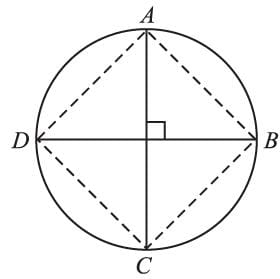∴ ABCD is a square.

Math Olympiad Test: Circles- 2 - Question 8

A is the center of the circle. ABCD is a parallelogram and CDE is a straight line. the ratio ∠DEB : ∠BCD is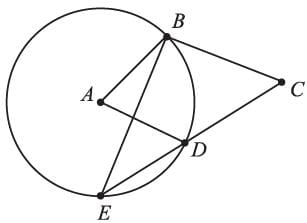Detailed Solution for Math Olympiad Test: Circles- 2 - Question 8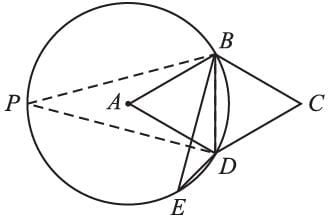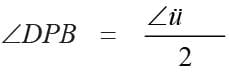(angle subtended at the center is double the angle at the circumference)
⇒ ∠BAD = 2∠DPB
∵ ABCD is a parallelogram
∴ ∠BAD = ∠BCD
⇒ ∠BCD = 2∠DPB
∠DEB = ∠BPD (angles in the same segment)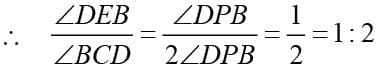Math Olympiad Test: Circles- 2 - Question 9

The radius of a circle is 26 cm and the length of the perpendicular from the center to the chord AB is equal to 10 cm. The length of AB is:

Detailed Solution for Math Olympiad Test: Circles- 2 - Question 9

According to the question,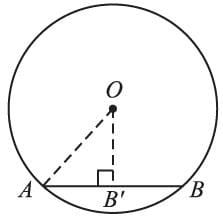OA = r = 26 cm
OB′ = 10 cm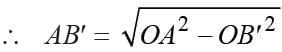(Using Pythagoras theorem)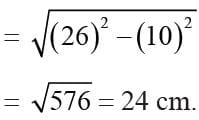∴ AB = 2 × AB′ = 2 × 24 cm = 48 cm.

Math Olympiad Test: Circles- 2 - Question 10

The radius of the circumcircle of an equilateral triangle having a length of each side equal to ‘a’ is:

Detailed Solution for Math Olympiad Test: Circles- 2 - Question 10

For an equilateral triangle ABC, O lies on the perpendicular from any vertex to the opposite side.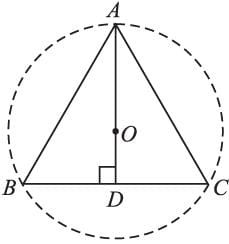Also,
AO : OD = 2 : 1 (for equilateral triangle)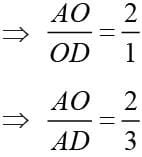(using componendo-dividendo)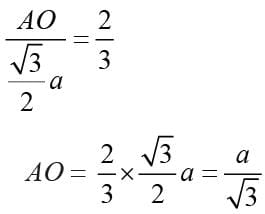Math Olympiad Test: Circles- 2 - Question 11

AB ≌ AC and O is the center of the circle, then,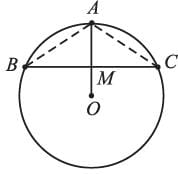Detailed Solution for Math Olympiad Test: Circles- 2 - Question 11

In ∆ABM and ∆ACM
AB ≅ AC (given)
AM = AM (common)
OM ⊥ BC (given)
∴ ∆ABM ≅ ∆ACM
∴  BM = CM

Math Olympiad Test: Circles- 2 - Question 12

AB and AC are two equal chords of a circle whose center is O. If AB ⊥ OD and OE ⊥ AC, then,

Detailed Solution for Math Olympiad Test: Circles- 2 - Question 12

In ∆ODE,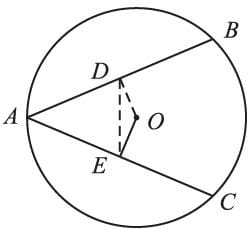OD = OE
∴ ∠ODE = ∠OED ……(i)
Now,
∠ODA = ∠ODE = 90° ….(ii)
Subtracting eq.(i) from (ii), we get
∠ODA – ∠ODE = ∠OEA – ∠OED
∴ AD = AE ⇒ ∆ADE is an isosceles triangle.

Math Olympiad Test: Circles- 2 - Question 13

In the adjoining figure, AB = AC and ∠ACB = 40°, then ∠BDC =?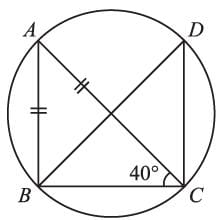Detailed Solution for Math Olympiad Test: Circles- 2 - Question 13

In ∆ABC,
AB = AC,
⇒ ∠ABC = ∠ACB = 40°
Also,
∠ABC + ∠BAC + ∠ACB = 180°
⇒ ∠BAC = 180° – 40° × 2
= 100°
∴ ∠BAC = ∠BDC = 100°
(angles in the same segment are equal).

Math Olympiad Test: Circles- 2 - Question 14

Chords AD and BC intersect each other at right angles at point P. If ∠DAB = 44°, then ∠ADC =?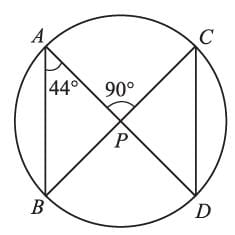Detailed Solution for Math Olympiad Test: Circles- 2 - Question 14

∵ ∠APC is an exterior angle for ∆ABP
∴ ∠ABP + ∠PAB = 90°
⇒ ∠ABP = 90° – 44° = 46°
(Angles in the same segment)
∴ ∠ABP = 46°

Math Olympiad Test: Circles- 2 - Question 15

If the length of an arc of a circle is proportional to the angle subtended by it at the center. Then, the ratio of ABC : circumference =?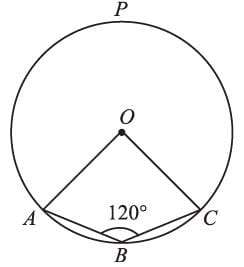Detailed Solution for Math Olympiad Test: Circles- 2 - Question 15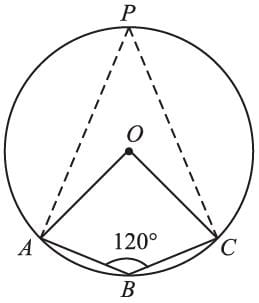∵ ABCP is a cyclic quadrilateral.
∴ ∠B + ∠P = 180°
⇒ ∠P = 180° – 120° = 60°
∠AOC = 2 ∠P = 2 × 60° = 120°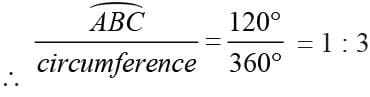## Mathematics Olympiad for Class 9

1 videos|43 tests
 Use Code STAYHOME200 and get INR 200 additional OFF Use Coupon Code
Information about Math Olympiad Test: Circles- 2 Page
In this test you can find the Exam questions for Math Olympiad Test: Circles- 2 solved & explained in the simplest way possible. Besides giving Questions and answers for Math Olympiad Test: Circles- 2, EduRev gives you an ample number of Online tests for practice

## Mathematics Olympiad for Class 9

1 videos|43 tests